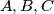### IMO Shortlist 1962 problem 5

Kvaliteta:
Avg: 0,0
Težina:
Avg: 0,0
On the circle$K$ there are given three distinct points$A,B,C$. Construct (using only a straightedge and a compass) a fourth point$D$ on$K$ such that a circle can be inscribed in the quadrilateral thus obtained.
Izvor: Međunarodna matematička olimpijada, shortlist 1962